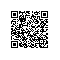# [ACM_水题] ZOJ 3706 [Break Standard Weight 砝码拆分，可称质量种类，暴力]

The balance was the first mass measuring instrument invented. In its traditional form, it consists of a pivoted horizontal lever of equal length arms, called the beam, with a weighing pan, also called scale, suspended from each arm (which is the origin of the originally plural term "scales" for a weighing instrument). The unknown mass is placed in one pan, and standard masses are added to this or the other pan until the beam is as close to equilibrium as possible. The standard weights used with balances are usually labeled in mass units, which are positive integers.

With some standard weights, we can measure several special masses object exactly, whose weight are also positive integers in mass units. For example, with two standard weights 1 and 5, we can measure the object with mass 145 or 6 exactly.

In the beginning of this problem, there are 2 standard weights, which masses are x and y. You have to choose a standard weight to break it into 2 parts, whose weights are also positive integers in mass units. We assume that there is no mass lost. For example, the origin standard weights are 4 and 9, if you break the second one into 4and 5, you could measure 7 special masses, which are 1, 3, 4, 5, 8, 9, 13. While if you break the first one into 1 and 3, you could measure 13 special masses, which are 1, 2, 3, 4, 5, 6, 7, 8, 9, 10, 11, 12, 13! Your task is to find out the maximum number of possible special masses.

#### Input

There are multiple test cases. The first line of input is an integer T < 500 indicating the number of test cases. Each test case contains 2 integers x and y. 2 ≤ xy ≤ 100

#### Output

For each test case, output the maximum number of possible special masses.

#### Sample Input

2
4 9
10 10


#### Sample Output

13
9


Author: YU, Zhi
Contest: The 10th Zhejiang Provincial Collegiate Programming Contest

 1 #include<iostream>
2 #include<algorithm>
3 #include<set>
4 using namespace std;
5 int main(){
6     int T;
7     cin>>T;
8     while(T--){
9         int x,y;
10         cin>>x>>y;
11         set<int>Q;
12         Q.clear();
13         int max=1;
14         for(int i=1;i<=x/2;i++){
15             int a=i,b=x-i,c=y;
16             Q.insert(a);//单独一个砝码
17             Q.insert(b);
18             Q.insert(c);
19             Q.insert(a+b);//2个砝码
20             if(a-b!=0)Q.insert(abs(a-b));
21             Q.insert(a+c);
22             if(a-c!=0)Q.insert(abs(a-c));
23             Q.insert(b+c);
24             if(b-c!=0)Q.insert(abs(b-c));
25             Q.insert(a+b+c);//3个砝码
26             if(a+b-c!=0)Q.insert(abs(a+b-c));
27             if(a-b+c!=0)Q.insert(abs(a-b+c));
28             if(a-b-c!=0)Q.insert(abs(a-b-c));
29             //cout<<a<<' '<<b<<' '<<c<<' '<<Q.size()<<'\n';
30             if(Q.size()>max)max=Q.size();
31             Q.clear();
32         }//拆x
33         for(int i=1;i<=y/2;i++){
34             int a=i,b=y-i,c=x;
35             Q.insert(a);
36             Q.insert(b);
37             Q.insert(c);
38             Q.insert(a+b);
39             if(a-b!=0)Q.insert(abs(a-b));
40             Q.insert(a+c);
41             if(a-c!=0)Q.insert(abs(a-c));
42             Q.insert(b+c);
43             if(b-c!=0)Q.insert(abs(b-c));
44             Q.insert(a+b+c);
45             if(a+b-c!=0)Q.insert(abs(a+b-c));
46             if(a-b+c!=0)Q.insert(abs(a-b+c));
47             if(a-b-c!=0)Q.insert(abs(a-b-c));
48             //cout<<a<<' '<<b<<' '<<c<<' '<<Q.size()<<'\n';
49             if(Q.size()>max)max=Q.size();
50             Q.clear();
51         }//拆y
52         cout<<max<<'\n';
53     }return 0;
54
55 }使用钉钉扫一扫加入圈子
+ 订阅